# Problems of the area of a circular sector

#### Number of problems found: 29

• Semicircle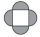The ornament consists of one square and four dark semicircles. The area of the square is 4 cm ^ 2. Find the area of one dark semicircle and round the result to hundreds.
• Goat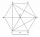The fenced flower bed has the shape of a regular hexagon. The tops are formed by fence posts. The fence around the flowerbed measures 60 m. A goat is tied to one of the pillars from the outside and grazes on the surrounding meadow (the goat should not ent
• A spherical segment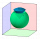The aspherical section, whose axial section has an angle of j = 120° in the center of the sphere, is part of a sphere with a radius r = 10 cm. Calculate the cut surface.
• Surface of the coneCalculate the surface of the cone if its height is 8 cm and the volume is 301.44 cm3.
• Volume of the coneCalculate the volume of the cone if the content of its base is 78.5 cm2 and the content of the shell is 219.8 cm2.
• Maximum of volumeThe shell of the cone is formed by winding a circular section with a radius of 1. For what central angle of a given circular section will the volume of the resulting cone be maximum?
• Sphere parts, segmentA sphere with a diameter of 20.6 cm, the cut is a circle with a diameter of 16.2 cm. .What are the volume of the segment and the surface of the segment?
• FlakesWe describe a circle of the square, and we describe a semicircle above each side of the square. This created 4 flakes. Which is bigger: the area of the central square, or the area of four flakes?
• Mice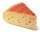Mice consumed a circular hole in a slice of cheese. The cheese has the shape of a circular cut with a radius of 20 cm and an angle of 90 degrees. What percentage of the cheese ate mice if they made 20 holes with a diameter of 2 cm?
• Three segments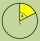The circle is divided into 3 segments. Segment A occupies 1/4 of the area. Segment B occupies 1/3 of the area. What part is occupied by section C? In what proportion are areas A: B: C?
• Quarter of a circleCalculate the circumference of a quarter circle if its content is S = 314 cm2.
• Irrigation sprinklerThe irrigation sprinkler can twist with an angle of 320° and has a reach of 12 meters. Which area can irrigate?
• Cylinder horizontallyThe cylinder with a diameter of 3 m and a height/length of 15 m is laid horizontally. Water is poured into it, reaching a height of 60 cm below the axis of the cylinder. How many hectoliters of water is in the cylinder?
• Circular segmentCalculate the area S of the circular segment and the length of the circular arc l. The height of the circular segment is 2 cm and the angle α = 60°. Help formula: S = 1/2 r2. (Β-sinβ)
• Equilateral triangle v3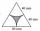Calculate the content of the colored gray part. Equilateral triangle has side length 8 cm. Arc centers are the vertices of a triangle.
• Math heart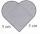Stylized heart shape created from a square with side 5 cm and two semicircles over his sides. Calculate the content area and its circumference.
• Arc-sectorarc length = 17 cm area of sector = 55 cm2 arc angle = ? the radius of the sector = ?
• Circular poolThe base of the pool is a circle with a radius r = 10 m, excluding a circular segment that determines the chord length 10 meters. The pool depth is h = 2m. How many hectoliters of water can fit into the pool?
• Arc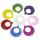What area of a circle occupied the flowers planted in the arc of a circle with radius 3 m with central angle 45°?
• Circle sectorThe circular sector with a central angle 160° has an area 452 cm2. Calculate its radius r.

Do you have an exciting math question or word problem that you can't solve? Ask a question or post a math problem, and we can try to solve it.

We will send a solution to your e-mail address. Solved examples are also published here. Please enter the e-mail correctly and check whether you don't have a full mailbox.

Circular sector Problems. Area - math problems.